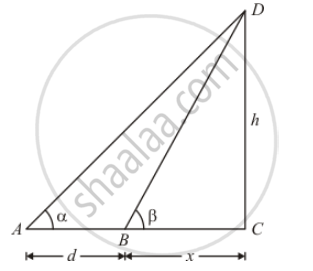Advertisement Remove all ads

# The Angle of Elevation of the Top of a Tower Standing on a Horizontal Plane from a Point a is α. After Walking a Distance D Towards the Foot of the Tower the Angle of Elevation is Found T - Mathematics

MCQ

The angle of elevation of the top of a tower standing on a horizontal plane from a point A is α. After walking a distance d towards the foot of the tower the angle of elevation is found to be β. The height of the tower is

#### Options

• $\frac{d}{cot \alpha + cot \beta}$

• $\frac{d}{cot \alpha + cot \beta}$

• $\frac{d}{\tan \beta - \tan \alpha}$

• $\frac{d}{\tan \beta - \tan \alpha}$

Advertisement Remove all ads

#### Solution

The given information can be represented with the help of a diagram as below.Here, CD = h is the height of the tower. Length of BC is taken as x.

InΔ ACD

tan A=(CD)/(AC)

tan∝=h/(d+x)

h=(d+x)tan∝ ............(1)

In ΔBCD.

tan ß = CD/BC

tan ß=h/x

x=h cot ß          ...............(2)

From (1) and (2)

h=(d+h cot ß)tan ∝

h=d tan ∝+h cot ß tan ∝

h(1-cot ß tan ∝ )= d tan ∝

h=d tan ∝/((1-cot ß tan ∝ ))=d/(cot ∝-cot ß)

Is there an error in this question or solution?
Advertisement Remove all ads

#### APPEARS IN

RD Sharma Class 10 Maths
Chapter 12 Trigonometry
Q 7 | Page 42
Advertisement Remove all ads

#### Video TutorialsVIEW ALL 

Advertisement Remove all ads
Share
Notifications

View all notifications

Forgot password?
Course